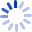Mathematical Methods in the Physical Sciences

Updates the original, comprehensive introduction to the areas of mathematical physics encountered in advanced courses in the physical sciences. Intuition and computational abilities are stressed. Original material on DE and multiple integrals has been expanded.

Cash for Textbooks